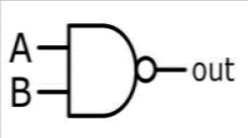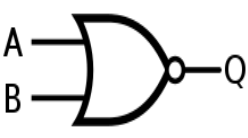# Digital Computer questions for Computer Science and MCA students

## Write a short note on System Software

• System software is a collection of programs designed to work with the hardware part of a system by acting as a link between the user and the hardware devices.
• It gets installed in the system along with the operating system and can further be updated.
• It works in the background.
• It is called a “low-level” software as the user has no idea about what tasks are being carried out by it and how.
Two types of the system softwares are

1. Operating Systems: Set of programs to manage the working of the computer hardware devices.
2. Utility: Any task which is performed for system management.
Example: Operating Systems make the best example for system softwares, like, LINUX, UNIX.

## Write a short notes on a) Universal Gates b) Assembler

a) Universal Gates
• The universal gate as the name suggests, are the ones which can generate any other logic gate. Using these gates any other gate can be obtained.
• NAND and NOR are termed as the universal gates as they can generate all the other gates. We will see how;
NAND Gate:

Below is the diagram for the NAND gate and seeing this diagram in parts it will be evident as to why it is called a universal gate.1. AND gate from NAND gate - If we see the two inputs A and B generating a single output, it explains how it has an AND gate in it.
2. NOT gate from NAND gate - Looking at the “knot” at the other end (the output part) makes it a NOT gate.
3. OR gate from NAND gate - Joining the two NAND gates will give us a OR gate

This explains how the three basic gates can be constructed using a NAND gate.

NOR Gate:

NOR gate is basically an inverted OR gate. It can be combined to make all other kinds of logic gates. Given below is a diagram of the “two input” NOR gate.Now we shall look into this diagram in parts, like we did earlier and see how other gates can be obtained from this.

1. OR gate from NOR gate: Its simply a NOR gate followed by a NOT gate.
2. AND gate from NOR gate: This can simply be done by inverting the outputs to a NOR gate.
3. NOT gate from NOR gate: This can simply be done by joining the two outputs of a NOR gate.

b) Assembler

The system cannot understand the code, programs or any instructions that are written and thus they have to be converted into the machine language to make it understandable.

Here is where an assembler is used. It takes the instructions and convert them into the language that the processors can understand and perform the operations. These instructions are termed as “assembly language”.

Basic functions of an assembler are
• It acts as an interpreter.
• It co-ordinates the developers to access the computer's hardware components.
• It also acts as a compiler of the assembly language.
They are divided into 2 types, namely

1. One pass assembler
2. Two pass assembler

This division of the assemblers into two types is concluded from the fact that how many times the assembler parses the code. The PASS1 assembler parse the code only once whereas the PASS2 assembler parse the code twice.